Mathematics
Easy

Question

# Estimate the difference of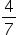and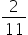by rounding to 0,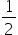, or 1.

## 01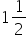Hint:

## The correct answer is:### In general, the whole can be any particular thing or value, and the fraction might be a portion of any quantity out of it. The top and bottom numbers of a fraction are explained by the fundamentals of fractions. Fractions are further broken down into various categories based on the characteristics of the numerator and denominator.As follows: Proper fractions Improper fractions Mixed fractions Like fractions Unlike fractions Equivalent fractions Estimating sums and differences of fractions to the nearest 1/2: Fractions < 1/4 are rounded down to 0 Fractions ≥ 1/4 and ≤ 3/4 are rounded to 1/2 Fractions > 3/4 are rounded up to 1 Here the fraction that we have given is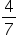and, we have to round them off near to 0,, or 1.So after looking at these numbers we can round them off as follows:can be rounded tocan be rounded to 0Now finding the difference, we get:- 0 =So, the difference is.

The numerical numbers that make up a fraction of the whole are called fractions. A whole can be a single item or a collection of items. A fraction is represented as a/b, where a and b are the numerator and denominator, respectively. Here we were given to find the difference between two fractional numbers after rounding them off, the final answer is.

### Related Questions to study#### With Turito Foundation.#### Get an Expert Advice From Turito.